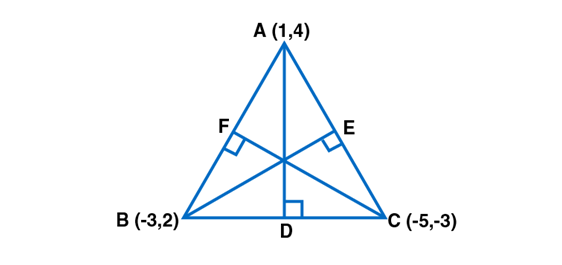• +91 9971497814
• info@interviewmaterial.com

# RD Chapter 23- The Straight Lines Ex-23.12 Interview Questions Answers

### Related Subjects

Question 1 : Find the equation of a line passing through the point (2, 3) and parallel to the line 3x– 4y + 5 = 0.

Given:

The equation isparallel to 3x − 4y + 5 = 0 and pass through (2, 3)

The equation of theline parallel to 3x − 4y + 5 = 0 is

3x – 4y+ λ = 0,

Where, λ isa constant.

It passes through (2,3).

Substitute the valuesin above equation, we get

3 (2) – 4 (3) + λ = 0

6 – 12 + λ =0

λ = 6

Now, substitute thevalue of λ = 6 in 3x – 4y + λ = 0, we get

3x − 4y + 6

The required line is3x − 4y + 6 = 0.

Question 2 : Find the equation of a line passing through (3, -2) and perpendicular to the line x – 3y + 5 = 0.

Given:

The equation isperpendicular to x – 3y + 5 = 0 and passes through (3,-2)

The equation of theline perpendicular to x − 3y + 5 = 0 is

3x + y + λ =0,

Where, λ isa constant.

It passes through(3, − 2).

Substitute the valuesin above equation, we get

3 (3) + (-2) +λ = 0

9 – 2 + λ =0

λ = – 7

Now, substitute thevalue of λ = − 7 in 3x + y + λ = 0, we get

3x + y – 7 = 0

The required line is3x + y – 7 = 0.

Question 3 : Find the equation of the perpendicular bisector of the line joining the points (1, 3) and (3, 1).

Given:

A (1, 3) and B (3, 1)be the points joining the perpendicular bisector

Let C be the midpointof AB.

So, coordinates of C =[(1+3)/2, (3+1)/2]

= (2, 2)

Slope of AB = [(1-3) /(3-1)]

= -1

Slope of theperpendicular bisector of AB = 1

Thus, the equation ofthe perpendicular bisector of AB is given as,

y – 2 = 1(x – 2)

y = x

x – y = 0

The required equationis y = x.

Question 4 : Find the equations of the altitudes of a ΔABC whose vertices are A (1, 4), B (-3, 2) and C (-5, -3).

Given:

The verticesof ∆ABC are A (1, 4), B (− 3, 2) and C (− 5, − 3).

Now let us find theslopes of ∆ABC.Slope of AB = [(2 – 4)/ (-3-1)]

= ½

Slope of BC = [(-3 –2) / (-5+3)]

= 5/2

Slope of CA = [(4 + 3)/ (1 + 5)]

= 7/6

Thus, we have:

Slope of CF = -2

Slope of BE = -6/7

Hence,

Equation of CF is:

y + 3 = -2(x + 5)

y + 3 = -2x – 10

2x + y + 13 = 0

y – 4 = (-2/5) (x – 1)

5y – 20 = -2x + 2

2x + 5y – 22 = 0

Equation of BE is:

y – 2 = (-6/7) (x + 3)

7y – 14 = -6x – 18

6x + 7y + 4 = 0

The requiredequations are 2x + y + 13 = 0, 2x + 5y – 22 = 0, 6x + 7y + 4 = 0.

Question 5 : Find the equation of a line which is perpendicular to the line√3x – y + 5 = 0 and which cuts off an intercept of 4 units with the negative direction of y-axis.

Given:

The equation isperpendicular to √3x – y + 5 = 0 equation and cuts off anintercept of 4 units with the negative direction of y-axis.

The line perpendicularto √3x – y + 5 = 0 is x + √3y + λ = 0

It is given that theline x + √3y + λ = 0 cuts off an intercept of 4 units with thenegative direction of the y-axis.

This means that theline passes through (0,-4).

So,

Let us substitute thevalues in the equation x + √3y + λ = 0, we get

0 – √3 (4) + λ = 0

λ = 4√3

Now, substitute thevalue of λ back, we get

x + √3y + 4√3 = 0

The required equationof line is x + √3y + 4√3 = 0.

Todays Deals### RD Chapter 23- The Straight Lines Ex-23.12 Contributorskrishan

Name:
Email:

# Latest News# 9000 interview questions in different categories